Get instant live expert help with Excel or Google Sheets“My Excelchat expert helped me in less than 20 minutes, saving me what would have been 5 hours of work!”

#### Post your problem and you’ll get expert help in seconds.

Your message must be at least 40 characters
Our professional experts are available now. Your privacy is guaranteed.

# Excel Sin Function – Excelchat

The SIN function in Excel determines the sine of any given angle in radians.

If our given angle is in degrees, we must first convert it to radians.

To supply our SIN degrees angle in radians, use the Excel RADIANS function to convert it, or multiply it by PI()/180.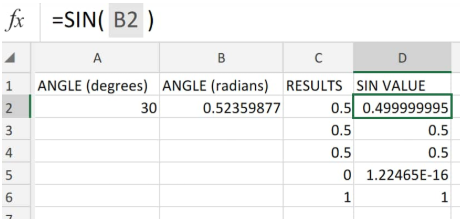Figure 1. of Sin Function in Excel

## Generic Formula

`=SIN (number)`

Where number = The angle – in radians – for which we want the sin in Excel.

The SIN function works in radians so the expected Excel sine value must be in radians.

## Converting sin degrees Excel to Radians

Since Excel sin angle value is in degrees, we will have to convert it to radians before we supply it to the sin function.

We can achieve this by using the Radians function in Excel as illustrated below;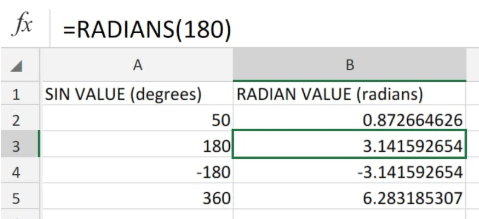Figure 2. of Converting Degrees to Radians in Excel

Notice that in our worksheet example above, 180 degrees converts into π radians while 360 degrees converts into 2π radians.

## How to Calculate Sine in Excel

Now that we are able to convert sine values to radians we can now demonstrate how to do sine in Excel.

1. Label the columns of our worksheet accordingly, and input the sin value for conversion;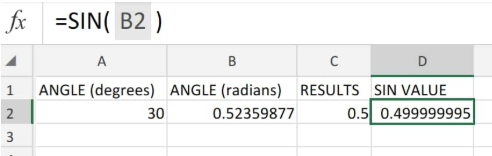Figure 3. of Sin Function in Excel

In the example displayed above, we initially converted the sin angle in cell A2 (30 degrees) to radians (cell B2).

We then obtained the sin of the resulting radian value by entering the following Excel Sine Function:

`=SIN(B2)`

## Note

We can also use other Excel math and trig functions such as cosine in Excel and the Excel Pi function to generate different results.

See illustration below;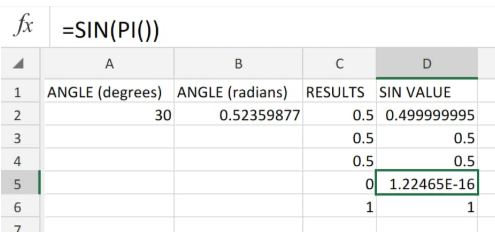Figure 4. of Sine Function in Excel

In cell D2, we used the Excel Pi function to generate the value.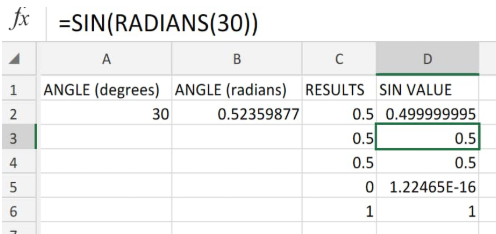Figure 5. of Sine Function in Excel

In cell D3, the Radians function in Excel is used to first convert the 30 degree angle into radians before being supplied to the Sine function.